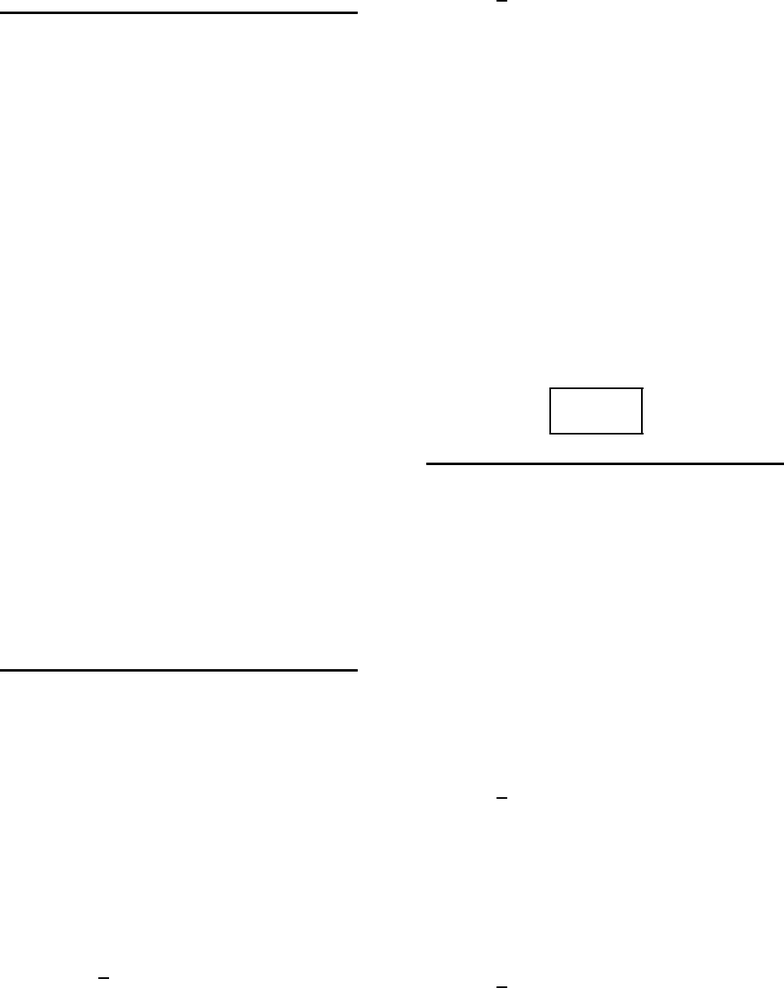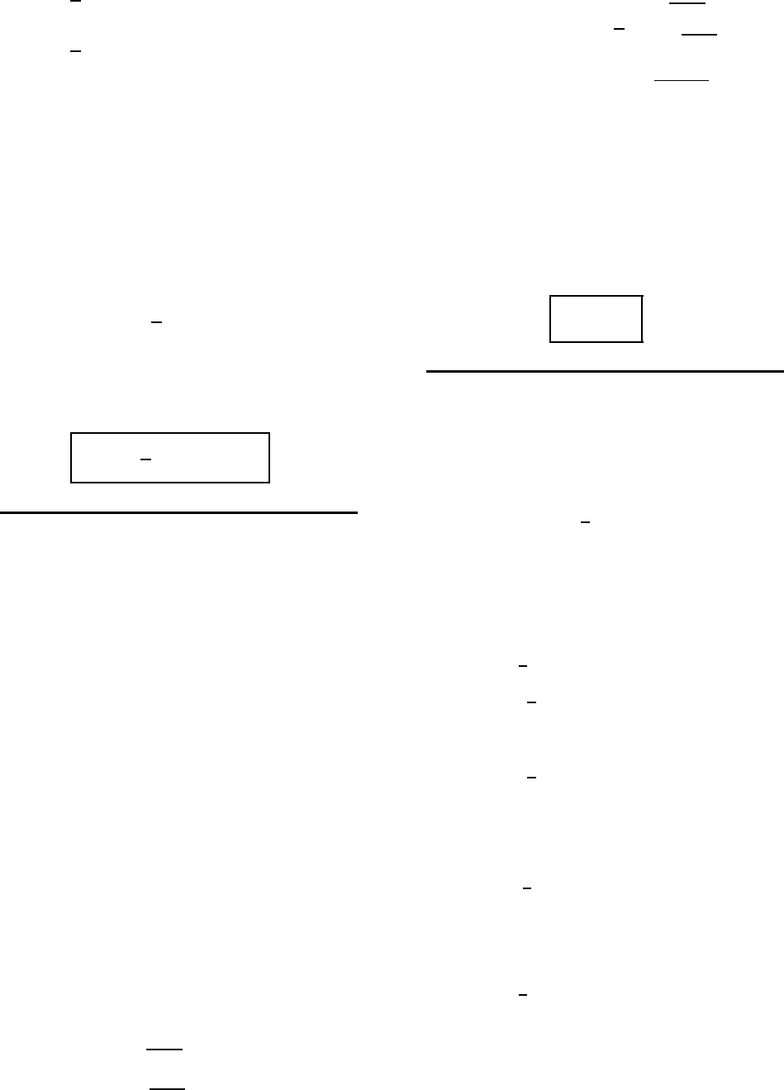# M 408D Chapter Notes - Chapter 15.2: Iterated Integral, Multiple Integral, Talking Lifestyle 1278

43 views6 pages
School
UT-Austin
Department
Mathematics
Course
M 408D
Professoroliver (smo998) – HW 15.2 – rusin – (52440) 1
This print-out should have 9 questions.
Multiple-choice questions may continue on
the next column or page – ﬁnd all choices
001 10.0 points
Which, if any, of the following are correct?
A. For all continuous functions g,
Z1
0Zy
0
g(x, y)dx dy =Z1
0Zx
0
g(x, y)dy dx.
B. For all continuous functions f,
Z1
0Z2
0
f(x, y)dx dy =Z2
0Z1
0
f(x, y)dx dy.
1. both of them
2. B only
3. neither of them correct
4. A only
Explanation:
A. False: incorrect reversal of the order of
integration when integrating over the upper
triangle in the square [0,1] ×[0,1].
B. False: incorrect reversal of the order of
integration when integrating over a rectangle.
002 10.0 points
Evaluate the iterated integral
I=Zπ
0Zcos(θ)
0
2esin(θ)dr dθ .
1. I=e2
2. I= 2e
3. I= 2 1
e1
4. I= 2(e1)
5. I= 0 correct
6. I=1
e2
Explanation:
After simple integration
Zcos(θ)
0
2esin(θ)dr =h2r esin(θ)icos(θ)
0
= 2 cos(θ)esin(θ).
In this case,
I=Zπ
0
2 cos(θ)esin(θ)=h2esin(θ)iπ
0.
Consequently,
I= 0 .
003 10.0 points
Evaluate the double integral
I=Z ZD
xsin(y)dxdy
when Dis the bounded region enclosed by the
graphs of
y= 0 , y =x2, x = 3 .
1. I=1
2(1 cos(9))
2. I= 9 cos(9)
3. I= 9 sin(9)
4. I= cos(9) 1
5. I=1
2(cos(9) 9)
6. I= sin(9) 1
Unlock document

This preview shows pages 1-2 of the document.
Unlock all 6 pages and 3 million more documents.oliver (smo998) – HW 15.2 – rusin – (52440) 2
7. I=1
2(sin(9) 1)
8. I=1
2(9 sin(9)) correct
Explanation:
After integration with respect to ywe see
that
I=Z3
0hxcos(y)ix2
0dx
=Z3
0
x(1 cos(x2)) dx
=1
2hx2sin(x2)i3
0,
using substitution in the second integral.
Consequently,
I=1
2(9 sin(9)) .
004 10.0 points
Evaluate the double integral
I=Z ZD
(3xy)dydx
where Dis the region bounded by the circle
with center at the origin and radius 1.
1. I= 0 correct
2. I=2
3. I=1
4. I=4
5. I=3
Explanation:
If we want to integrate ﬁrst with respect to
y, the double integral becomes
I=Z1
1Z1x2
1x2
(3xy)dydx .
But then
I=Z1
1h3xy 1
2y2i1x2
1x2
= 6 Z1
1
xp1x2dx .
Using substitution we thus see that
I= 2h1x23/2i1
1.
Consequently,
I= 0 .
005 10.0 points
Reverse the order of integration in the inte-
gral
I=Z9
3 Z1
x/3
f(x, y)dy!dx,
but make no attempt to evaluate either inte-
gral.
1. I=Z1
3/3 Z3y2
3
f(x, y)dx!dy
2. I=Z3/3
0 Z9x2
3
f(x, y)dx!dy
3. I=Z3/3
0Z3
9y2
f(x, y)dxdy
4. I=Z1
3/3 Z9y2
3
f(x, y)dx!dy cor-
rect
5. I=Z1
3/3Z3
3y2
f(x, y)dxdy
Explanation:
The region of integration is similar to the
one in the ﬁgure
Unlock document

This preview shows pages 1-2 of the document.
Unlock all 6 pages and 3 million more documents.

## Document Summary

Oliver (smo998) hw 15. 2 rusin (52440) Multiple-choice questions may continue on the next column or page nd all choices before answering. Which, if any, of the following are correct: for all continuous functions g, 0 g(x, y) dy dx: i = 0 correct, i = 2 esin( ) dr = h 2 r esin( )icos( ) = 2 cos( ) esin( ) : for all continuous functions f , 0 f (x, y) dx dy =z 2. 0 f (x, y) dx dy: both of them, b only, neither of them correct, a only. 2 cos( ) esin( ) d = h 2 esin( )i . 10. 0 points: false: incorrect reversal of the order of integration when integrating over the upper triangle in the square [0, 1] [0, 1], false: incorrect reversal of the order of integration when integrating over a rectangle.